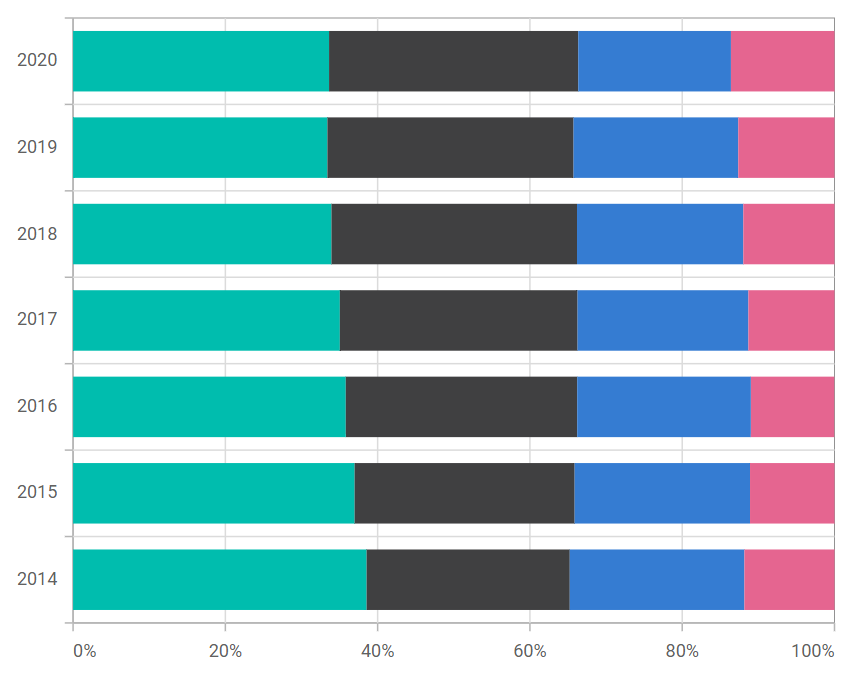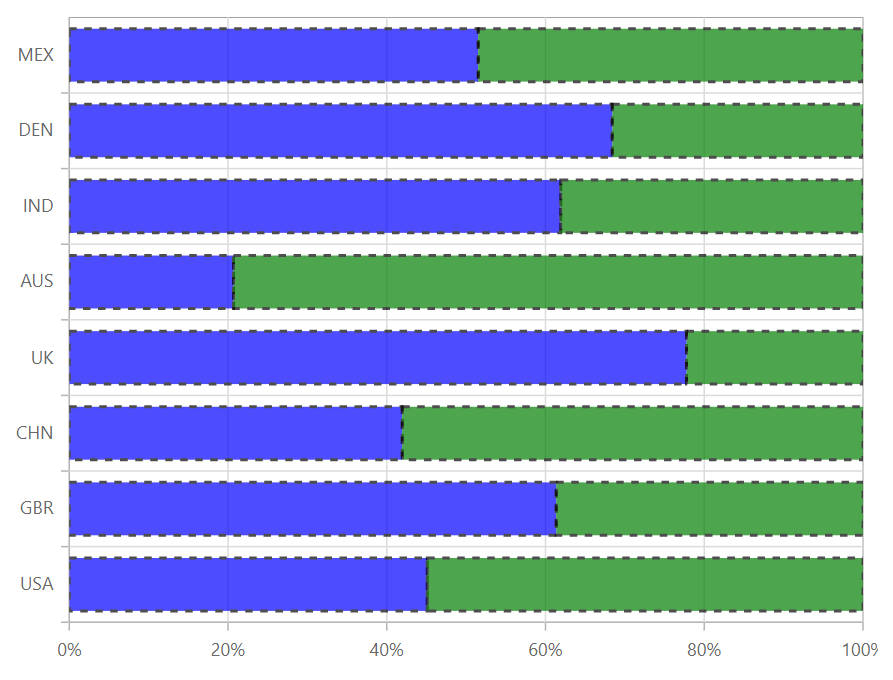# 100% Stacked Bar in Blazor Charts Component

17 Nov 20234 minutes to read

## 100% Stacked Bar

100% Stacked Bar Chart displays multiple series of data as stacked bars, ensuring that the cumulative proportion of each stacked element always totals 100%. Hence, the y-axis will always be rendered with the range 0–100%. To render a 100% Stacked Bar series, set the series Type as StackingBar100.

``````@using Syncfusion.Blazor.Charts

<SfChart>
<ChartPrimaryXAxis ValueType="Syncfusion.Blazor.Charts.ValueType.Category"></ChartPrimaryXAxis>

<ChartSeriesCollection>
<ChartSeries DataSource="@MedalDetails" XName="X" YName="YValue" Type="ChartSeriesType.StackingBar100">
</ChartSeries>
<ChartSeries DataSource="@MedalDetails" XName="X" YName="YValue1" Type="ChartSeriesType.StackingBar100">
</ChartSeries>
</ChartSeriesCollection>
</SfChart>

@code{
public class ChartData
{
public string X { get; set; }
public double YValue { get; set; }
public double YValue1 { get; set; }
}

public List<ChartData> MedalDetails = new List<ChartData>
{
new ChartData { X= "USA", YValue= 46, YValue1=56 },
new ChartData { X= "GBR", YValue= 27, YValue1=17 },
new ChartData { X= "CHN", YValue= 26, YValue1=36 },
new ChartData { X= "UK", YValue= 56,  YValue1=16 },
new ChartData { X= "AUS", YValue= 12, YValue1=46 },
new ChartData { X= "IND", YValue= 26, YValue1=16 },
new ChartData { X= "DEN", YValue= 26, YValue1=12 },
new ChartData { X= "MEX", YValue= 34, YValue1=32},
};
}``````NOTE

Refer to our Blazor 100% Stacked Bar Chart feature tour page to know about its other groundbreaking feature representations. You can also explore our Blazor 100% Stacked Bar Chart Example to know how to render and configure the 100% Stacked Bar type chart.

## Series customization

The following properties can be used to customize the 100% Stacked Bar series.

``````@using Syncfusion.Blazor.Charts

<SfChart>
<ChartPrimaryXAxis ValueType="Syncfusion.Blazor.Charts.ValueType.Category"></ChartPrimaryXAxis>

<ChartSeriesCollection>
<ChartSeries DataSource="@MedalDetails" XName="X" YName="YValue" DashArray="5,5" Fill="blue" Opacity="0.7" Type="ChartSeriesType.StackingBar100">
<ChartSeriesBorder Width="2" Color="black"></ChartSeriesBorder>
</ChartSeries>
<ChartSeries DataSource="@MedalDetails" XName="X" YName="YValue1" DashArray="5,5" Fill="green" Opacity="0.7" Type="ChartSeriesType.StackingBar100">
<ChartSeriesBorder Width="2" Color="black"></ChartSeriesBorder>
</ChartSeries>
</ChartSeriesCollection>
</SfChart>

@code{
public class ChartData
{
public string X { get; set; }
public double YValue { get; set; }
public double YValue1 { get; set; }
}

public List<ChartData> MedalDetails = new List<ChartData>
{
new ChartData { X= "USA", YValue= 46, YValue1=56 },
new ChartData { X= "GBR", YValue= 27, YValue1=17 },
new ChartData { X= "CHN", YValue= 26, YValue1=36 },
new ChartData { X= "UK", YValue= 56,  YValue1=16 },
new ChartData { X= "AUS", YValue= 12, YValue1=46 },
new ChartData { X= "IND", YValue= 26, YValue1=16 },
new ChartData { X= "DEN", YValue= 26, YValue1=12 },
new ChartData { X= "MEX", YValue= 34, YValue1=32},
};
}``````NOTE

Refer to our Blazor Charts feature tour page for its groundbreaking feature representations and also explore our Blazor Chart Example to know various chart types and how to represent time-dependent data, showing trends at equal intervals.